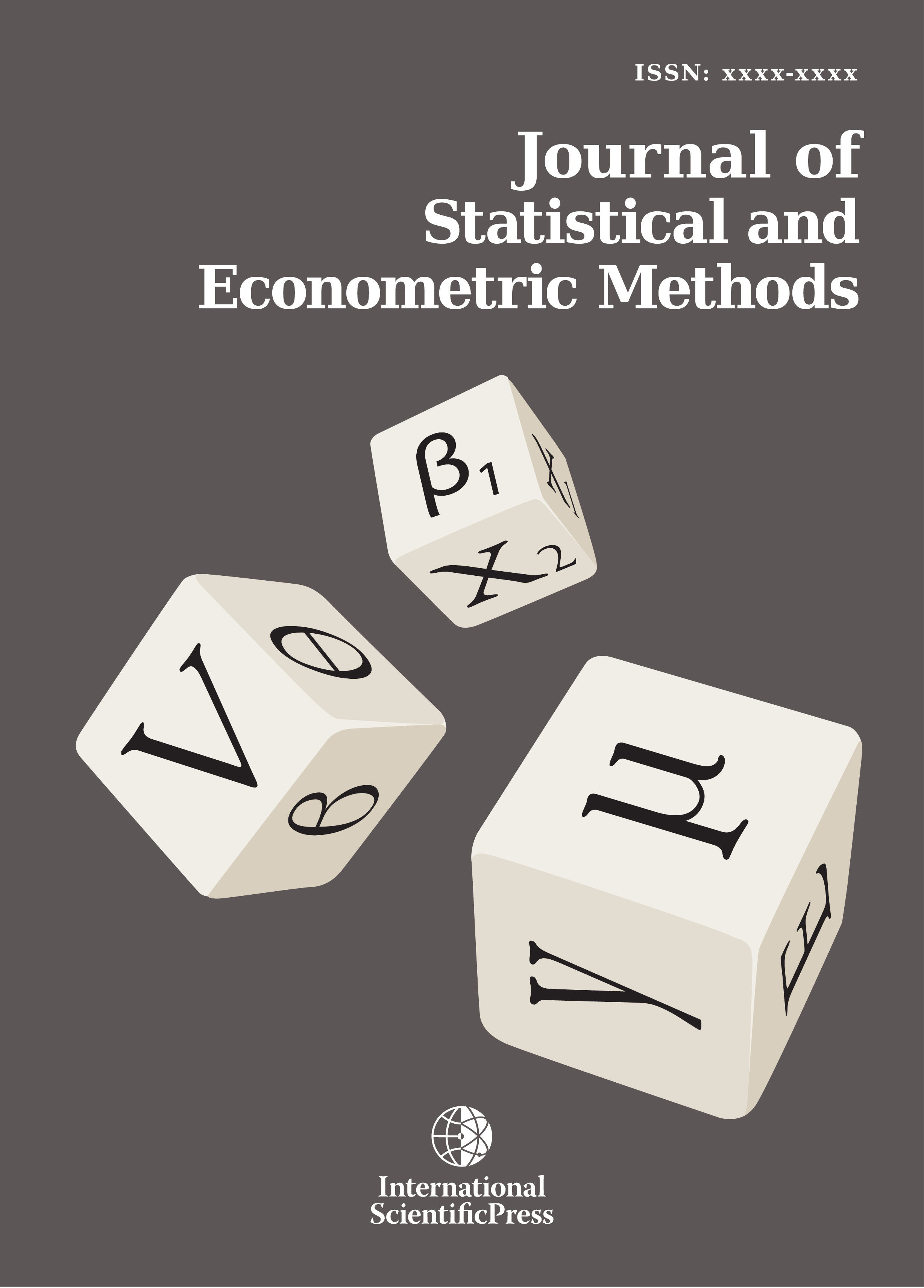# Journal of Statistical and Econometric Methods

#### Upper Bounds for Ruin Probability in a Controlled Risk Process under Rates of Interest with Homogenous Markov Chains

•[ Download ]
• Times downloaded: 385
• Abstract

This paper explores recursive and integral equations for ruin probability of a controlled risk process under rates of interest with homogenous Markov chains. We assume that claim and rates of interest are homogenous Markov chains, take a countable number of non – negative values. Generalized Lundberg inequalities for ruin probability of this process are derived via a recursive technique. Recursive equations for finite time ruin probability and an integral equation for ultimate ruin probability are presented, from which corresponding probability inequalities and upper bounds are obtained. An illustrative numerical example is discussed.

Mathematics Subject Classification: 62P05, 60G40, 12E05
Keywords: ruin probability, homogenous Markov chain, controlled risk processISSN: 2241-0376 (Online)
2241-0384 (Print)